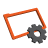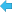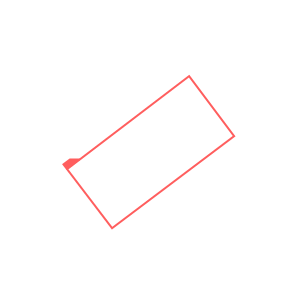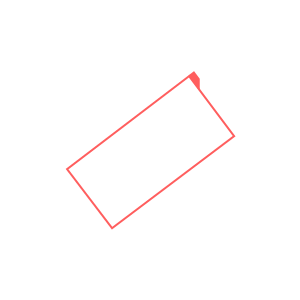Back to Adaptive Vision Studio website# NormalizeRectangleOrientation

Changes orientation of the given rectangle according to parameters.

Name Type DescriptioninRectangle Rectangle2D Input rectangleinReferenceAngle Real The middle angle of the valid range of the output rectangle's angleinRectangleOrientation RectangleOrientation Orientation of the output rectangleoutRectangle Rectangle2D

### Description

The operation changes the input rectangle's marked corner so the output rectangle's rotation angle is the lowest possible not lower than inReferenceAngle - 90 satisfying the inRectangleOrientation parameter at the same time. Some sample parameters configurations are:
• inReferenceAngle = 90, inRectangleOrientation = Any – the marked corner will have the least y coordinate possible
• inReferenceAngle = 0, inRectangleOrientation = Any – the marked corner will have the least x coordinate possible
• inRectangleOrientation = Horizontal – the output rectangle's width will be not shorter than its height
• inRectangleOrientation = Vertical – the output rectangle's height will be not shorter than its width

### Examples

Description of usage of this filter can be found in examples and tutorial: Badge Measurement, Button Presence.NormalizeRectangleOrientation performed on a sample rectangle with inReferenceAngle = 90 and inRectangleOrientation = Any.

### Complexity Level

This filter is available on Basic Complexity Level.